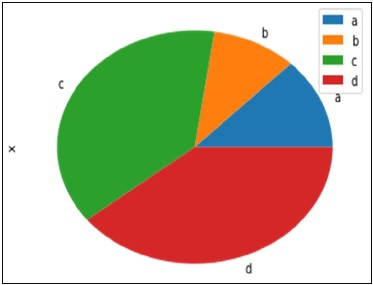# Python Pandas - Visualization

### Basic Plotting: plot

This functionality on Series and DataFrame is just a simple wrapper around the matplotlib libraries plot() method.

```import pandas as pd
import numpy as np

df = pd.DataFrame(np.random.randn(10,4),index=pd.date_range('1/1/2000',
periods=10), columns=list('ABCD'))

df.plot()
```

Its output is as follows −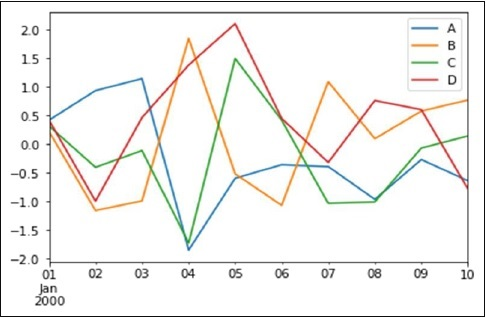If the index consists of dates, it calls gct().autofmt_xdate() to format the x-axis as shown in the above illustration.

We can plot one column versus another using the x and y keywords.

Plotting methods allow a handful of plot styles other than the default line plot. These methods can be provided as the kind keyword argument to plot(). These include −

• bar or barh for bar plots
• hist for histogram
• box for boxplot
• 'area' for area plots
• 'scatter' for scatter plots

## Bar Plot

Let us now see what a Bar Plot is by creating one. A bar plot can be created in the following way −

```import pandas as pd
import numpy as np

df = pd.DataFrame(np.random.rand(10,4),columns=['a','b','c','d')
df.plot.bar()
```

Its output is as follows −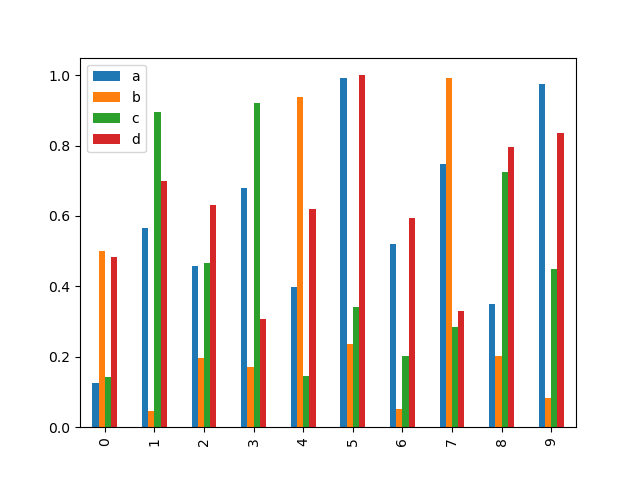To produce a stacked bar plot, pass stacked=True

```import pandas as pd
df = pd.DataFrame(np.random.rand(10,4),columns=['a','b','c','d')
df.plot.bar(stacked=True)
```

Its output is as follows −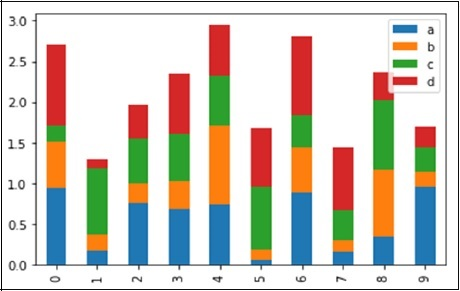To get horizontal bar plots, use the barh method −

```import pandas as pd
import numpy as np

df = pd.DataFrame(np.random.rand(10,4),columns=['a','b','c','d')

df.plot.barh(stacked=True)
```

Its output is as follows −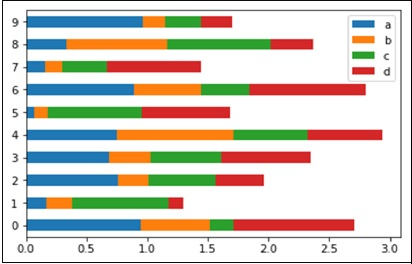## Histograms

Histograms can be plotted using the plot.hist() method. We can specify number of bins.

```import pandas as pd
import numpy as np

df = pd.DataFrame({'a':np.random.randn(1000)+1,'b':np.random.randn(1000),'c':
np.random.randn(1000) - 1}, columns=['a', 'b', 'c'])

df.plot.hist(bins=20)
```

Its output is as follows −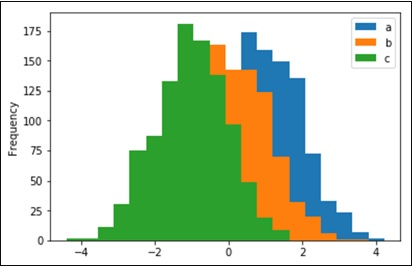To plot different histograms for each column, use the following code −

```import pandas as pd
import numpy as np

df=pd.DataFrame({'a':np.random.randn(1000)+1,'b':np.random.randn(1000),'c':
np.random.randn(1000) - 1}, columns=['a', 'b', 'c'])

df.diff.hist(bins=20)
```

Its output is as follows −## Box Plots

Boxplot can be drawn calling Series.box.plot() and DataFrame.box.plot(), or DataFrame.boxplot() to visualize the distribution of values within each column.

For instance, here is a boxplot representing five trials of 10 observations of a uniform random variable on [0,1).

```import pandas as pd
import numpy as np
df = pd.DataFrame(np.random.rand(10, 5), columns=['A', 'B', 'C', 'D', 'E'])
df.plot.box()
```

Its output is as follows −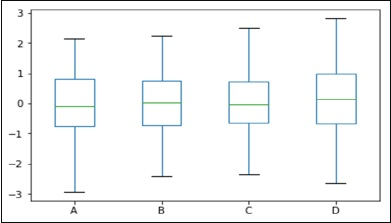## Area Plot

Area plot can be created using the Series.plot.area() or the DataFrame.plot.area() methods.

```import pandas as pd
import numpy as np

df = pd.DataFrame(np.random.rand(10, 4), columns=['a', 'b', 'c', 'd'])
df.plot.area()
```

Its output is as follows −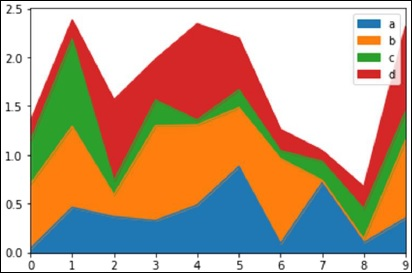## Scatter Plot

Scatter plot can be created using the DataFrame.plot.scatter() methods.

```import pandas as pd
import numpy as np
df = pd.DataFrame(np.random.rand(50, 4), columns=['a', 'b', 'c', 'd'])
df.plot.scatter(x='a', y='b')
```

Its output is as follows −## Pie Chart

Pie chart can be created using the DataFrame.plot.pie() method.

```import pandas as pd
import numpy as np

df = pd.DataFrame(3 * np.random.rand(4), index=['a', 'b', 'c', 'd'], columns=['x'])
df.plot.pie(subplots=True)
```

Its output is as follows −